﻿ The Universal Gravitational VariablePublications are Open
Access in this journal
Article Versions
Export Article
• Normal Style
• MLA Style
• APA Style
• Chicago Style
Research Article
Open Access Peer-reviewed

### The Universal Gravitational Variable

José Luís Pereira Rebelo Fernandes
International Journal of Physics. 2020, 8(1), 35-38. DOI: 10.12691/ijp-8-1-6
Received March 03, 2020; Revised April 10, 2020; Accepted April 19, 2020

### Abstract

Local cosmological phenomena, such as the systematic and constant Moon distancing of 3.82+-0.07 cm, per year, from the Earth, Ref. ” informed by NASA, and the systematic distancing of the 60 moons of Jupiter and 40 moons of Saturn from its planets, “Ref. ”, and the constant value of the velocity of light “C”, as well, the variation of time in the inverse square root ratio of Universal density of potential Energy at local, “Ref. ”, requires a review of the local universe and the laws govern. The value of the Universal Gravitational Constant is called into question, concluding that it is variable and that its value is proportional to the expansion of the universe, that is, inversely proportional to the universal density of potential energy at the local. In the solar system, the increasing of the distance of the moons relative to their planets is only possible if G-Universal Gravitational Constant increases in the same proportion as this increase in distance, this increase at a constant value of G is proportional to the systematic and constant removal of the Moon from Earth. To understand the reason for the increase in G, we look at the Constant Velocity of Light propose by Einstein and we conclude that the increase in G is inversely proportional to the Universal Density of Potential Energy at local and is inversely proportional to the expansion of the Universe, then G is proportional to the expansion of the Universe. If G is proportional to the increase at a constant value of the gravitational radius, and proportional to the expansion of the Universe, then the Universal expansion also occurs at a constant value. (This study will be based on our Earth reference and considering it with actual characteristics, such as mass, time, speed of light, etc.)

### 1. The Relativity of the Time with the Universal Density of Potential Energy at Different Stationary Reference Frame 

Through the analysis of Einstein's relativity derived from the Schwarzschild metric, it is proposed to vary the time between the reference frame A within the gravitational field of the mass M, and another reference frame C outside the gravitational field, given by: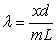(1.1)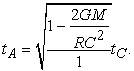(2.1)

Multiplying the numerator and denominator in Eq. (2.2) by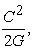we have: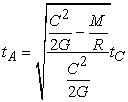(3.1)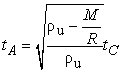(4.1)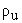- Universal density of potential energy, generated by all the universal masses, at A,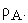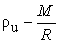- Universal density of potential energy, generated by all the universal masses, we have to subtract the influence of the Earth on its surface,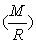at C,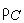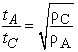(5.1)

Yes, the time is inversely proportional to the square root of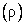the Universal Density of Potential Energy al local.

The universal density of potential energy varies from one location to another location.

The time in each location varies in the inverse proportion of the square root of the respective universal density of potential energy.

With universal expansion the universal density of potential energy decreases (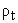) and will cause the contraction of the time, too in the Earth reference.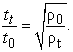(6.1)

### 2. The Local Universe

2.1. The Constant Velocity of Light

There is a data in the local universe that has come to us through Einstein, the constancy of the "speed of light" C in all directions. This is the maximum speed allowed in any direction of space. We are in the presence of local maximum escape potential, given by:

At where:– Universal density of potential energy at in place, generated at the locality by all the universal masses.

G – "Universal gravitational constant"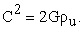As postulated, C constant.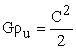(1.2)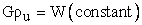(2.2)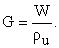(3.2)

In an expanding Universe, with the removal of all masses from the location, we will have in time: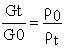(4.2)

G is inversely proportional to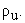G is no more than the coefficient of the gravity radiation capacity through the vacuum, through theThe lower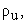the lower the resistance to radiation propagation through the void, causing G to increase in inverse proportion.

We have a Universal Gravitational Variable and not a Constant.

But why will G be variable and increasing?

2.2. G and ρu

In universal terms:

Einstein characterized the speed of light as a result of the escape potential anywhere and in all directions.

The same happens in the local escape potential where the escape velocity to abandon a mass is the same in all directions.

In gravitational potential,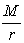is the density of potential energy created by mass M at distance r. Since we are facing a universal escape potential,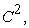thencan only be the density of potential energy created by all the universal mass in the place.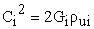(5.2)

Where: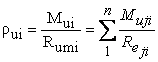(6.2)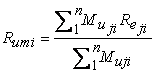- The Average distance to which is found as universal that creates density of potential energy in location.

The amount of universal mass / energy will always be constant.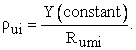(7.2)

In an expanding universe, all the universal masses will be more and more distant from location i, so the average radius of universal mass emission to the site will be increasing.

If the average radius of radiation increases, then the local density of universal potential energy decreases.

On the other hand, as we have seen, and according to Eq. (3.2):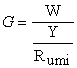(8.2)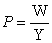(9.2)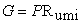(10.2)

G increases in proportion to the increase of the average universal emission radius, then, G will also grow at the ratio of the average radius of universal mass emission to the local. Because we are in a homogeneous Universe, then we can say that G increases in proportion to the expansion of the Universe.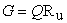(11.2)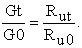(12.2)

We have a Universal Gravitational Variable and not a Constant.

### 3. Local Gravitational Field

With the available information that the Moon is moving away from Earth, at a constant rate of 3.82 cm per year, ”Ref. 14“, obtained as measured since 1969, i.e. measurements taken for over 51 years, through the Apollo Laser Ranging Experiments Yield Results, and “Jupiter has more than 60 natural satellites, but only the top four deserve particular attention: Io, Europe, Ganymede and Callisto. They have nearly circular orbits, and exhibit the same face toward Jupiter. They are also slowly moving away from the planet. Saturn has over forty satellites, except for two, always run with the same face toward the planet, and they are slowly moving away."(Extracted from the book "Discovering the Universe", PhD Teresa Lago from the Astrophysics Center of the University of Porto), “Ref. 14”.

These phenomena require an analysis of the local universe and the laws that govern it.

It is known by all, the expression that allows us to calculate the gravitational potential of a body around the other: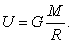(1.3)

The gravitational potential will always be constant. (Constant velocity of the gravitational bodies in void U=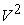): A body in the void, in a null working system is always constant. U= Constant.

The generanting mass of the gravitational field will also remain constant, solving Eq.(1.3): M= Constant.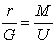(2.3)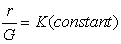(3.3)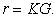(4.3)

In an expanding Universe, with the removal of all masses from the location, we will have in time:.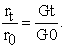(5.3)

As we have seen before, bodies belonging to a gravitational field are moving away from the masses that generate these fields. In the Earth / Moon System, the Moon is moving away from the Earth every year, that is, the radius of gravitation increases,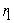>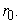The same happens with the moons of Jupiter and Saturn. From Eq. (5.3), we know that the gravitational radius increases indicating that G increases proportionally to the radius.

Increasing G, we have the same gravitational potential, generated the largest distance of mass M and hence the Moon to adjust to the location of this potential, moving away.

As the radius of gravitation increases to the constant value, then G will also increase to the constant value as it is proportional to it.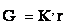(6.3)

Since the Moon's annual distance from Earth is constant, so the change in G will also be constant.

We have a Universal Gravitational Variable and not a Constant.

### 4. The Space Vacuum

We find through the various reviews already carried out that the universal space is permeated by the universal density of potential energy resulting from mass radiation. We also find that the gravitational radiation also depends on the universal density of the potential energy in the site, the lower the Universal Density of potential energy, the lower the resistance to the propagation of the gravitational waves making G greater. This type of radiation is non-corpuscular, and our devices that, like us, are materials will not be able to detect them directly.

This concept was already implied by Einstein when he made the potential;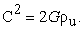### 5. The Cosmological Variable

Given the proposed expression for the cosmological constant: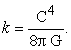(1.5)

Since G varies, then we will not have a cosmological constant but a cosmological variable whose value will vary in the inverse proportion of the universal gravitational variable.

### 6. The Expansion of the Universe Don’t Need "dark energy"

Once the expansion of the universe has begun with the Big Bang, it will never be blocked because the universal gravitational variable will grow in proportion to this expansion, thus guaranteeing the universe's gravitational and expansionary stability.

The universe expands, locally the density of universal potential energy decreases, which increases the universal gravitational variable in place, allowing the masses to move away from the mass center of the gravitational field without changing their velocities because the same potentials will happen the longest distance.

The process is interactive and the universe keeps stable.

So the Universe, from the point of view of the constant gravitational potential created, will behave in the same way as a "static universe" and will not collapse.

Now we don’t need any dark energy to explain the expanding universe.

“Dark energy” isn’t more than the increase in Universal Gravitational Variable.

### 7. Conclusion

In the local Universe with the information that the Moon is moving away from the Earth, a constant 3.82cm per year and that the Moons of Jupiter and Saturn are moving away from the respective planets, we conclude that the gravitational radius is proportional to G and that the gravitational radius are increasing at constant value, then G is also increasing at constant value like the Universal expansion.

(I think that the remoteness of the remaining moons from their planets also occurs at constant value, as one day will prove).

Though moving away, the Moon will always remain in the gravitational field of Earth. (Only a cataclysm would change such)

In an attempt to understand the reason for the increase in G, we look at the General Universe and conclude that the constancy of the speed of light is only possible if the G variation happens in the inverse proportion of the value of the Local Universal Density of Potential Energy,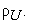These observations make us accept the existence of the Universal Gravitational Variable and not the Constant Gravitational Variable, being inversely proportional toAt a lower local universal density of potential energy we find a higher universal gravitational variable at local, that is, a greater capacity of radiation transmission in space.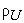decreases due to the expansion of the universe. The farther away are the universal masses of a given place, the lower the potential created by them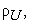will thus be inversely proportional to this expansion.

Now we know that in our homogeneous universe, the part will grow in the same proportion of the whole.

Through the analysis of a black hole it is concluded that radiation that promotes universal density of potential energy as well the gravity, which propagate at velocity C does not curve under the action of local matter.

The universal emptiness is permeated by the universal density potential energy, resulting from mass radiation, which radiation such as gravitational radiation is non-corpuscular in nature and thus not detectable directly by our apparatus of a corpuscular nature. Its detection will only be possible indirectly and through the fluctuation of G or ofThe universe grows at speed C. The universe grows at constant value.

Now we do not need any dark energy to explain the expanding universe. The "dark energy" is no more than the increase of the gravitational variable.

Now we have the cosmological variable and not the cosmological constant.

The weight of matter in each of the stars will increase due to the increase of G.

The escape velocity of the stars will tend to increase. Will it ever be harder to leave Earth? What we have to do sooner or later, to preserve the species.

In all planetary systems, galaxies, clusters of galaxies, superclusters of galaxies are moving away from their centers of gravity. This is universal growth.

Every planet in the solar system is moving away from the Sun, at least as long as the loss of its mass is less than the expansion rate of the Universe.

All natural moons are moving away from their planets.

The Sun departs every year from the Center of the Milky Way.

The planets in the past of the planetary system have been closer to their stars. Mars has been as far from the Sun as Earth is now. Venus will soon be as far from the Sun as Earth is now.

How was the moon created? Was there a single double planet with two centers of mass in which the increase of G caused the centers of mass to move away, thereby "tearing out" the Moon, the single Earth protrusion of the surface layer of Earth, always keeping the same face facing the Earth?

Considering that, The Magnetic Permeability of the Vacuum will increase like G, then, applying quantum mechanics, would we conclude that the entire atomic radius will tend to decrease causing the shrinking of stars and planets. This would allow a better understanding of how universal structures were formed?

This increase in G leads us to think that in the past G would be much smaller and as such we will have to change our dates?

### References

  José Luís Pereira Rebelo Fernandes, The Relativity of the Time with the Universal Density of Potential Energy at Different Stationary Reference Frames, International Journal of Physics. 2020, 8(1), 11-13. In article  Selleri, F, Lessons of relativity, from Eisntein to the ether of Lorentz, Edições Duarte Reis e Franco Selleri, 2005, 227. In article  Deus, J.D, Pimenta, M, Noronha, A, Peña, T and Brogueira, Introdução à Física, Introduction to Physics, Mc. Graw-Hill, 2000, 633. In article  Eisberg, R, Resnick, , Quantum Physics of atoms, molecules, solids, nuclei, and particles, Elsevier, 1979, 928 In article  Crawford, Paulo. «A Teoria da Relatividade e o 'Global Positioning System' (GPS)» (PDF). Universidade de Lisboa. Consultado em 18 de fevereiro de 2016. Cópia arquivada (PDF) em 5 de novembro de 2013. In article  Taylor, J.H., “Binary Pulsars and Relativistic Gravity”, Rev. Mod. Phys., 66, 711-719, (1994). In article View Article  J. Natário, General Relativity Without Calculus, Springer (2011). In article View Article  PubMed  C. Rousseau e Y. Saint-Aubin, Mathematics and Technology, Springer (2008) In article View Article  E. Taylor and J. Wheeler, Exploring Black Holes: Introduction to General Relativity, Addison Wesley (2000). In article  M.G.M. de Magalhães, D. Schiel, I.M. Guerrini e E. Marega Jr., Revista Brasileira de Ensino de Física 24, 97 (2002). In article View Article  E.C. Ricardo, J.F. Custódio e M.F. Rezende Jr., Revista Brasileira de Ensino de Física 29, 137 (2007). https://eclipse.gsfc.nasa.gov/SEhelp/ApolloLaser.html. In article View Article  Lunar laser ranging: the millimeter challenge, T W Murphy, Rep. Prog. Phys.76 (2013) https://tmurphy.physics.ucsd.edu/papers/rop-llr.pdf. In article View Article  PubMed  Lunar Geophysics, Geodesy, and Dynamics, James G. Williams and Jean O. Dickey, 2002 https://ilrs.cddis.eosdis.nasa.gov/docs/williams_lw13.pdf. In article  Lago, Teresa Descobrir o Universo, Gradiva, junho de 2006. In articleThis work is licensed under a Creative Commons Attribution 4.0 International License. To view a copy of this license, visit http://creativecommons.org/licenses/by/4.0/